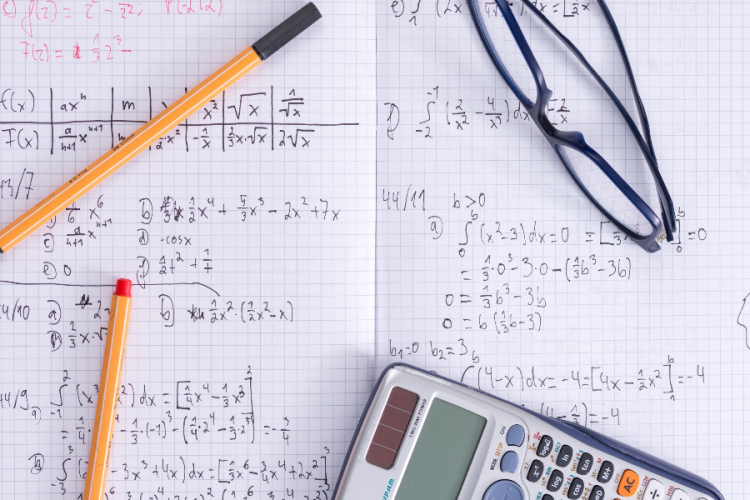# Different methods of solving various mathematical equationsIn both mathematics and physics, equations are extremely significant. Equations are used to solve questions in Kinematics, Torque, Thermodynamics, and many other relevant topics. Chemistry makes use of equations as well. This is why understanding the various approaches to dealing with equations is critical. Equations are used to solve problems involving interest, percentages, profit and loss, solving quadratic equations and a variety of other topics. The different methods for solving systems of equations are discussed in this article. It will also educate readers on the significance of equations.

## Solving system of equations:

• Simultaneous equation solution: This method is only applicable to equations with two variables. By multiplying both equations with a common factor, one of the variables is eliminated in this procedure. The value of a single variable is then determined by dividing the equation’s value by the quantity in front of the variable. The other variable’s value is derived by plugging the first variable’s value into the equation. This strategy is taught to students in lower grades, and many questions about it are posed in important exams.
• Matrices: A system of three variables can be simply solved using the matrices approach. To reach the result, one must calculate the adjoint of the matrix. This technique is used to address difficult problems. This strategy produces reliable findings and is simple to comprehend. It is necessary to understand the fundamental concepts of matrices to solve equations. The Gauss Jordan Method, the Gauss Elimination Method, and the Gauss Siedel Method are only a few examples. Using the concept of Matrices, all of these methods are applied to solve the equations. If students have a rudimentary understanding of Matrices, they can readily solve issues involving equations.